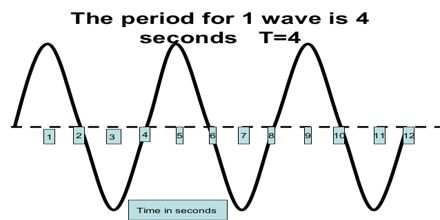Physics

# Determining Wave FrequencyPrinciple objective of this lecture is to present on how determine Wave Frequency from a graph. It is a tutorial based lecture. Frequency, also called wave frequency, is a measurement of the total number of vibrations or oscillations made within a certain amount of time. Wavelength is also measured in metres (m) – it is a length after all. The frequency, f, of a wave is the number of waves passing a point in a certain time. We normally use a time of one second, so this gives frequency the unit hertz (Hz), since one hertz is equal to one wave per second.